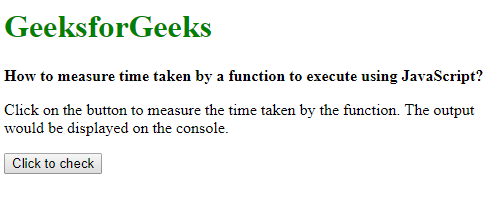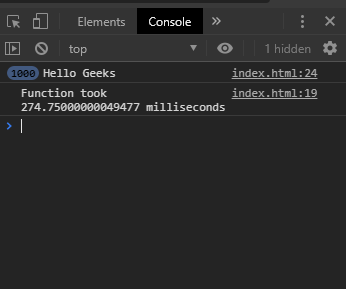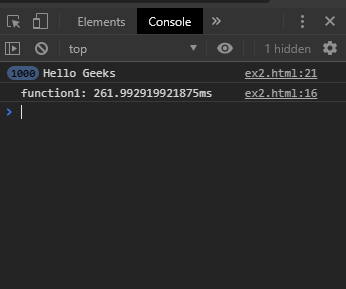# How to measure time taken by a function to execute using JavaScript?

Method 1: Using the performance.now() method: The now() method of the performance interface returns a high-resolution timestamp whenever it is called during the program. The time can be measured by getting the starting time before the function and ending time after the function and then subtracting both of them. This gives the time elapsed for the function.

Syntax:

```start = performance.now();
function_to_call();
end = performance.now();
```

Example:

 ` ` `<``html``> ` `     `  `<``head``> ` `    ``<``title``> ` `        ``How to measure time taken by a function ` `        ``to execute using JavaScript? ` `    `` ` ` ` ` `  `<``body``> ` `    ``<``h1` `style``=``"color: green"``> ` `        ``GeeksforGeeks ` `    `` ` `     `  `    ``<``b``> ` `        ``How to measure time taken by a function ` `        ``to execute using JavaScript? ` `    `` ` `     `  `    ``<``p``> ` `        ``Click on the button to measure the time ` `        ``taken by the function. The output would ` `        ``be displayed on the console. ` `    `` ` `     `  `    ``<``button` `onclick``=``"measurePerformance()"``> ` `        ``Click to check ` `    `` ` `     `  `    ``<``script` `type``=``"text/javascript"``> ` `        ``function measurePerformance() { ` `            ``start = performance.now(); ` `            ``exampleFunction(); ` `            ``end = performance.now(); ` `            ``timeTaken = end - start; ` `            ``console.log("Function took " + ` `                    ``timeTaken + " milliseconds"); ` `        ``} ` ` `  `        ``function exampleFunction() { ` `            ``for (i = 0; i < ``1000``; i++) { ` `                ``console.log('Hello Geeks'); ` `            ``} ` `        ``} ` `    `` ` ` ` ` `  `                     `

Output:

• Before clicking the button:• After clicking the button:Method 2: Using console.time() method: The time() and timeEnd() methods of Console object could be used as a timer to measure the time taken between these two methods. It takes a label parameter that could be used to distinguish between multiple timers. Whenever the timeEnd() method is called, the timer is stopped and the time output is given to the console.

Syntax:

```console.time('label');
function_to_call();
console.timeEnd('label');
```

Example:

 ` ` `<``html``> ` `     `  `<``head``> ` `    ``<``title``> ` `        ``How to measure time taken by a function ` `        ``to execute using JavaScript? ` `    `` ` ` ` ` `  `<``body``> ` `    ``<``h1` `style``=``"color: green"``> ` `        ``GeeksforGeeks ` `    `` ` `     `  `    ``<``b``> ` `        ``How to measure time taken by a function ` `        ``to execute using JavaScript? ` `    `` ` `     `  `    ``<``p``> ` `        ``Click on the button to measure the time ` `        ``taken by the function. The output would ` `        ``be displayed on the console. ` `    `` ` `     `  `    ``<``button` `onclick``=``"measurePerformance()"``> ` `        ``Click to check ` `    `` ` `     `  `    ``<``script` `type``=``"text/javascript"``> ` `        ``function measurePerformance() { ` `            ``console.time('function1'); ` `            ``exampleFunction(); ` `            ``console.timeEnd('function1'); ` `        ``} ` ` `  `        ``function exampleFunction() { ` `            ``for(i= 0;i <``1000``; i++) { ` `                ``console.log('Hello Geeks'); ` `            ``} ` `        ``} ` `    `` ` ` ` ` `  `                     `

Output:

• Before clicking the button:• After clicking the button:My Personal Notes arrow_drop_upIm listening

If you like GeeksforGeeks and would like to contribute, you can also write an article using contribute.geeksforgeeks.org or mail your article to contribute@geeksforgeeks.org. See your article appearing on the GeeksforGeeks main page and help other Geeks.

Please Improve this article if you find anything incorrect by clicking on the "Improve Article" button below.